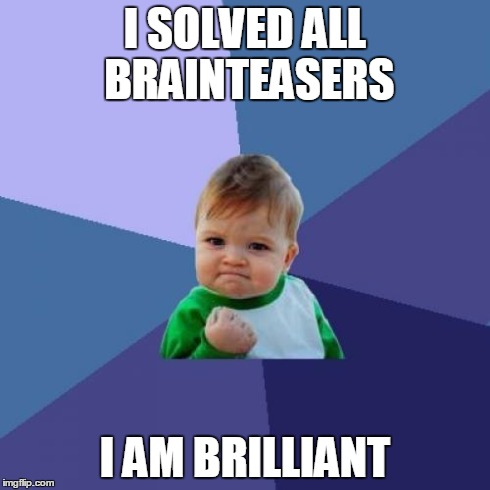# Coding puzzles & Quiz Winners

Thank you again for the participation in our programming quiz. We hope you enjoyed it! We reviewed all the entries and are happy to announce winners. Winners are Smahi, Neha, Suresh and Mena. Please congratulate them for solving the coding puzzles correctly.

In addition, in this blog we present even more coding puzzles in C# for you to practice and improve your learning process. Please find them below. Please write answers in the comments bellow and we will be happy to review them.Can you solve these coding puzzles? Can you describe the algorithm?

1). Puzzle 1

for (int p = 0; p < 4; p++)
{
for (int q = 0; q < 4; q++)
{
Console.Write(p * q);
}
}
2). Puzzle 2
for (int p = 0; p < 4; p++)
{
for (int q = 0; q < 4 – p; q++)
{
Console.Write(p – q);
}
}
3). Puzzle 3
int[] a = { 9, 8, 1, 10, 4, 6, 5, -1, 3, 2 };
int t = a;
for (int i = 0; i < 9; i++)
{
if (t > a[i])
{
t = a[i];
}
}
Console.Write(t);
4). Puzzle 4
float j = 2, k = 2;
for (int i = 0; i < 100000; i++)
{
k = k / 2;
j += k;
}
Console.Write(j);
//Puzzle 5
for (int i = 0; i < 10; i++)
{
if (Test(i)) Console.Write(i);
}
}
5). Puzzle 5
for (int i = 0; i < 10; i++)
{
if (Test(i)) Console.Write(i);
}
}
// Puzzle 5 Method
static bool Test(int number)
{
for (int i = 2; i < number; i++)
{
if (number % i == 0)
{
return false;
}
}
return true;
}
Scroll to Top